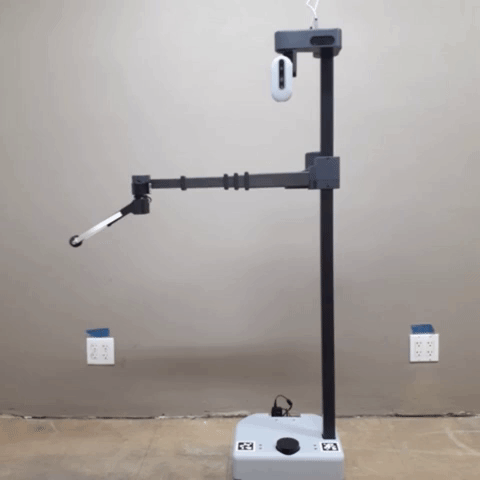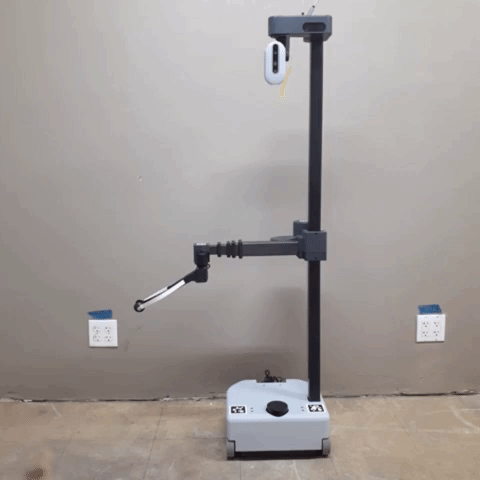## FollowJointTrajectory Commands

Note

ROS 2 tutorials are still under active development.

Stretch driver offers a `FollowJointTrajectory` action service for its arm. Within this tutorial, we will have a simple FollowJointTrajectory command sent to a Stretch robot to execute.

## Stow Command ExampleBegin by launching stretch_driver in a terminal.

``````ros2 launch stretch_core stretch_driver.launch.py
``````

In a new terminal type the following commands.

``````ros2 run stretch_ros_tutorials stow_command
``````

This will send a FollowJointTrajectory command to stow Stretch's arm.

### The Code

``````#!/usr/bin/env python3
import rclpy
from rclpy.node import Node
from rclpy.duration import Duration
from rclpy.action import ActionClient
import sys
from control_msgs.action import FollowJointTrajectory
from trajectory_msgs.msg import JointTrajectoryPoint
from sensor_msgs.msg import JointState
class StowCommand(Node):
def __init__(self):
super().__init__('stretch_stow_command')
self.joint_state = JointState()
self.trajectory_client = ActionClient(self, FollowJointTrajectory, '/stretch_controller/follow_joint_trajectory')
server_reached = self.trajectory_client.wait_for_server(timeout_sec=60.0)
if not server_reached:
self.get_logger().error('Unable to connect to arm action server. Timeout exceeded.')
sys.exit()
self.subscription = self.create_subscription(JointState, '/stretch/joint_states', self.joint_states_callback, 1)
self.subscription
def joint_states_callback(self, joint_state):
self.joint_state = joint_state
def issue_stow_command(self):
joint_state = self.joint_state
if (joint_state is not None):
self.get_logger().info('stowing...')
stow_point1 = JointTrajectoryPoint()
stow_point2 = JointTrajectoryPoint()
duration1 = Duration(seconds=0.0)
duration2 = Duration(seconds=4.0)
stow_point1.time_from_start = duration1.to_msg()
stow_point2.time_from_start = duration2.to_msg()
joint_value1 = joint_state.position # joint_lift is at index 1
joint_value2 = joint_state.position # wrist_extension is at index 0
joint_value3 = joint_state.position # joint_wrist_yaw is at index 8
stow_point1.positions = [joint_value1, joint_value2, joint_value3]
stow_point2.positions = [0.2, 0.0, 3.14]
trajectory_goal = FollowJointTrajectory.Goal()
trajectory_goal.trajectory.joint_names = ['joint_lift', 'wrist_extension', 'joint_wrist_yaw']
trajectory_goal.trajectory.points = [stow_point1, stow_point2]
self.trajectory_client.send_goal_async(trajectory_goal)
self.get_logger().info('Sent stow goal = {0}'.format(trajectory_goal))
def main(args=None):
rclpy.init(args=args)

stow_command = StowCommand()

rclpy.spin_once(stow_command)
stow_command.issue_stow_command()
rclpy.spin(stow_command)
stow_command.destroy_node()
rclpy.shutdown()
if __name__ == '__main__':
main()
``````

### The Code Explained

Now let's break the code down.

``````#!/usr/bin/env python3
``````
Every Python ROS Node will have this declaration at the top. The first line makes sure your script is executed as a Python script.

``````import rclpy
from rclpy.node import Node
from rclpy.duration import Duration
from rclpy.action import ActionClient
import sys
from control_msgs.action import FollowJointTrajectory
from trajectory_msgs.msg import JointTrajectoryPoint
from sensor_msgs.msg import JointState
``````

You need to import rclpy if you are writing a ROS 2 Node. Import the FollowJointTrajectory from the control_msgs.msg package to control the Stretch robot. Import JointTrajectoryPoint from the trajectory_msgs package to define robot trajectories.

``````class StowCommand(Node):
def __init__(self):
super().__init__('stretch_stow_command')
``````

The `StowCommand` class inherits from the `Node` class from and is initialized.

``````def issue_stow_command(self):
``````

The `issue_stow_command()` method will stow Stretch's arm. Within the function, we set stow_point as a `JointTrajectoryPoint`and provide desired positions (in meters). These are the positions of the lift, wrist extension, and yaw of the wrist, respectively. These are defined in the next set of the code.

``````    stow_point1 = JointTrajectoryPoint()
stow_point2 = JointTrajectoryPoint()
duration1 = Duration(seconds=0.0)
duration2 = Duration(seconds=4.0)
stow_point1.time_from_start = duration1.to_msg()
stow_point2.time_from_start = duration2.to_msg()
joint_value1 = joint_state.position # joint_lift is at index 1
joint_value2 = joint_state.position # wrist_extension is at index 0
joint_value3 = joint_state.position # joint_wrist_yaw is at index 8
stow_point1.positions = [joint_value1, joint_value2, joint_value3]
stow_point2.positions = [0.2, 0.0, 3.14]
trajectory_goal = FollowJointTrajectory.Goal()
trajectory_goal.trajectory.joint_names = ['joint_lift', 'wrist_extension', 'joint_wrist_yaw']
trajectory_goal.trajectory.points = [stow_point1, stow_point2]
``````

Set trajectory_goal as a `FollowJointTrajectory.Goal()` and define the joint names as a list. Then `trajectory_goal.trajectory.points` is defined by the positions set in stow_point. Specify the coordinate frame that we want (base_link) and set the time to be now.

``````    self.trajectory_client.send_goal_async(trajectory_goal)
self.get_logger().info('Sent stow goal = {0}'.format(trajectory_goal))
``````
Make the action call and send the goal.

``````def main(args=None):
rclpy.init(args=args)

stow_command = StowCommand()

rclpy.spin_once(stow_command)
stow_command.issue_stow_command()
rclpy.spin(stow_command)
stow_command.destroy_node()
rclpy.shutdown()
``````

Create a funcion, `main()`, to do all of the setup in the class and issue the stow command. Initialize the `StowCommand()` class and set it to node and run the `main()` function.

``````if __name__ == '__main__':
main()
``````

To make the script executable call the main() function like above.

## Multipoint Command ExampleIf you have killed the above instance of stretch_driver relaunch it again through the terminal.

``````ros2 launch stretch_core stretch_driver.launch.py
``````

In a new terminal type the following commands.

``````ros2 run stretch_ros_tutorials multipoint_command
``````

This will send a list of JointTrajectoryPoint's to move Stretch's arm.

### The Code

``````#!/usr/bin/env python3
import sys
import rclpy
from rclpy.node import Node
from rclpy.action import ActionClient
from rclpy.duration import Duration
from control_msgs.action import FollowJointTrajectory
from trajectory_msgs.msg import JointTrajectoryPoint
from sensor_msgs.msg import JointState
class MultiPointCommand(Node):
def __init__(self):
super().__init__('stretch_multipoint_command')
self.trajectory_client = ActionClient(self, FollowJointTrajectory, '/stretch_controller/follow_joint_trajectory')
server_reached = self.trajectory_client.wait_for_server(timeout_sec=60.0)
if not server_reached:
self.get_logger().error('Unable to connect to arm action server. Timeout exceeded.')
sys.exit()
self.subscription = self.create_subscription(JointState, '/stretch/joint_states', self.joint_states_callback, 1)
self.subscription
self.get_logger().info('issuing multipoint command...')
def joint_states_callback(self, joint_state):
self.joint_state = joint_state
def issue_multipoint_command(self):
joint_state = self.joint_state
duration0 = Duration(seconds=0.0)
duration1 = Duration(seconds=2.0)
duration2 = Duration(seconds=4.0)
duration3 = Duration(seconds=6.0)
duration4 = Duration(seconds=8.0)
duration5 = Duration(seconds=10.0)

joint_value1 = joint_state.position # joint_lift is at index 1
joint_value2 = joint_state.position # wrist_extension is at index 0
joint_value3 = joint_state.position # joint_wrist_yaw is at index 8
point0 = JointTrajectoryPoint()
point0.positions = [joint_value1, joint_value2, joint_value3]
point0.velocities = [0.2, 0.2, 2.5]
point0.accelerations = [1.0, 1.0, 3.5]
point0.time_from_start = duration0.to_msg()
point1 = JointTrajectoryPoint()
point1.positions = [0.3, 0.1, 2.0]
point1.time_from_start = duration1.to_msg()
point2 = JointTrajectoryPoint()
point2.positions = [0.5, 0.2, -1.0]
point2.time_from_start = duration2.to_msg()
point3 = JointTrajectoryPoint()
point3.positions = [0.6, 0.3, 0.0]
point3.time_from_start = duration3.to_msg()
point4 = JointTrajectoryPoint()
point4.positions = [0.8, 0.2, 1.0]
point4.time_from_start = duration4.to_msg()
point5 = JointTrajectoryPoint()
point5.positions = [0.5, 0.1, 0.0]
point5.time_from_start = duration5.to_msg()
trajectory_goal = FollowJointTrajectory.Goal()
trajectory_goal.trajectory.joint_names = ['joint_lift', 'wrist_extension', 'joint_wrist_yaw']
trajectory_goal.trajectory.points = [point0, point1, point2, point3, point4, point5]
self.trajectory_client.send_goal_async(trajectory_goal)
self.get_logger().info('Sent stow goal = {0}'.format(trajectory_goal))
def main(args=None):
rclpy.init(args=args)
multipoint_command = MultiPointCommand()
rclpy.spin_once(multipoint_command)
multipoint_command.issue_multipoint_command()
rclpy.spin(multipoint_command)
multipoint_command.destroy_node()
rclpy.shutdown()
if __name__ == '__main__':
main()
``````

### The Code Explained.

Seeing that there are similarities between the multipoint and stow command nodes, we will only breakdown the different components of the multipoint_command node.

``````        point1 = JointTrajectoryPoint()
point1.positions = [0.3, 0.1, 2.0]
point1.time_from_start = duration1.to_msg()
``````

Set point1 as a `JointTrajectoryPoint`and provide desired positions (in meters). These are the positions of the lift, wrist extension, and yaw of the wrist, respectively.

Note

The lift and wrist extension can only go up to 0.2 m/s. If you do not provide any velocities or accelerations for the lift or wrist extension, then they go to their default values. However, the Velocity and Acceleration of the wrist yaw will stay the same from the previous value unless updated.

``````        trajectory_goal = FollowJointTrajectory.Goal()
trajectory_goal.trajectory.joint_names = ['joint_lift', 'wrist_extension', 'joint_wrist_yaw']
trajectory_goal.trajectory.points = [point0, point1, point2, point3, point4, point5]
Set trajectory_goal as a `FollowJointTrajectory.Goal()` and define the joint names as a list. Then `trajectory_goal.trajectory.points` is defined by a list of the 6 points. Specify the coordinate frame that we want (base_link) and set the time to be now.